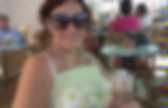# Maths

## Fractions

Mathantics. MooMoo Math and Science. Fractions. M & M Addition and Subtraction. Long Division. Symmetry & Transformation. Data Handling. Japanese crossword #10495. Free Multiplication Games, Activities, & Math Resources. Multiplication Strategies. Illustrated Mathematics Dictionary. A Maths Dictionary for Kids 2014 by Jenny Eather. Patterns. Free Math Videos for Kids - Numbers, Shapes, Fun Video Clips Online. Ordered Pairs and Coordinate Plane Worksheets. Ordered Pairs and Plotting Points Identifying Position: Real life Objects Identify the position of each item placed on the coordinate grid.Also find the item located at given ordered pairs. Only Positive: Sheet 1 | Sheet 2 | Sheet 3 All Quadrants: Sheet 1 | Sheet 2 | Sheet 3 Identifying Ordered Pairs There are two sections in each worksheet. Time Zone Map. DST – Click red dot for more info Legend: How to use the interactive Time Zone Map Search for any city in the search field above and place a black "pin" by that city on the map.If you place more than one pin, an extra line of information is added underneath the map with links to those cities' pages. You may add as many pins as you like. To remove the pins permanently (undo not possible), simply click here or on the Time Zone Map link in the menu above.Hover your mouse over any of the red dots to see the name of the city and country and current local time. More about the Time Zone Map. Question Database. Mathopolis. Angles - Acute, Obtuse, Straight and Right. An angle measures the amount of turn Names of Angles As the Angle Increases, the Name Changes: Try It Yourself: Also: the letter "A" has an acute angle.Be Careful What You Measure. Angles - Acute, Obtuse, Straight and Right. Cool Math 4 Kids - Geometry and Cool Math Art (fractals, tessellations, polyhedra and more) Math Play - Free Online Math Games. Free Math Dictionary, Free Math Dictionary for Kids, Free Math Dictionaries online - iCoachMath. Free maths worksheets. A Maths Dictionary for Kids 2013 by Jenny Eather. Free maths worksheets. Maths Worksheets. Categories in Maths Worksheets We have arranged all our Maths Worksheets into the following categories.Each has many resources within so why not jump in and explore the site? Popular Maths Worksheets Our most popular Maths Worksheets. Each of these resources has been selected due to the number of times they have been viewed by our members. Counting gorillas 1 to 5 These great, coloured pages will help your child to count up to 5 and become confident with small numbers. View 4 pages Using 2D shape (1) Here we have some great pages which will help your child to recognise the four basic 2-D shapes: circles, squares, rectangles and triangles.

Add to 10p Add the 10p coin to the other coins to find out how much the rabbit has. 5 pages Using the Add Sign Pirates Jolly pirates introduce the add sign for sums with numbers up to 5. Add 3 small numbers (1) Use the number line to add three small numbers. Addition Brainwhizz (1) Abacus for Numbers up to 99 Subtract a single digit from 2 digits. Measurement Worksheets. Multiplication Timed Test. Free Multiplication Games, Activities, & Resources. Multiplication Timed Test. Free Math Dictionary, Free Math Dictionary for Kids, Free Math Dictionaries online - iCoachMath. Maths Zone. Cool math 4 kids .com. IXL Math. Free, printable math resources. In this section of the site you will find multiplication tables, place value charts, decimal charts, a large quantity of different number lines, fraction bars, printable flashcards, and much more.All the resources are free and formatted for quick download and easy printing.You can also take any of them and modify them and reuse them on your own web site or web page. What is in the Printables section? Welcome to Math Playground. Geometry games and resources online. Table of Contents - Math Open Reference. Dad's Worksheets. This page contains links to free math worksheets for Basic Geometry problems.Click one of the buttons below to view a worksheet and its answer key. You can also use the 'Worksheets' menu on the side of this page to find worksheets on other math topics. Complementary Angle Subtraction Subtraction practice for finding complementary angles (Angles whose sum is 90 degrees). 3-D Shapes. Sixth Grade Math. 6th Grade Math First Grade Second Grade Third Grade Fourth Grade Fifth Grade Sixth Grade GeometryAngles Review Math Worksheet: Classify this angle as right, acute or obtuse: Estimating Angles Math Worksheet: Estimate the measure of the angle: Parallel, Perpendicular, Intersecting Lines Math Worksheet: If angle X = 132, then angle Y =Worksheets. Quadrilaterals and Polygons Worksheets Here is a graphic preview for all of the Quadrilaterals and Polygons Worksheets Sections. You can select different variables to customize these Quadrilaterals and Polygons Worksheets for your needs. The Quadrilaterals and Polygons Worksheets are randomly created and will never repeat so you have an endless supply of quality Quadrilaterals and Polygons Worksheets to use in the classroom or at home. Shapechallenge. Miss Mason's Shape Challenge How many of my challenges can you complete?You may need to follow the HELP onto the Internet to get your answers. Complete as many as you can then save the page under your name. What is a 3D shape? Complete the table about 2D shapes Number of sides Number of angles Examples of Shape Complete the table about 3D shapes Shape Number of faces Number of edges Number of vertices Examples of Shape. Laura Candler's Math File Cabinet. Math is Fun - Maths Resources. KS2 Bitesize - Maths.

Untitled. Free Math Worksheets: generate math worksheets for basic operations, fractions, decimals, measuring, clock, percents, number charts, square roots, and factoring. Math and Science For Children - Pre - K , Kindergarten to 7th Grades. Math is Fun - Maths Resources. Interaktive math.